# Math in Focus Grade 8 Chapter 1 Lesson 1.2 Answer Key Exponential Notation

Go through the Math in Focus Grade 8 Workbook Answer Key Chapter 1 Lesson 1.2 The Product and the Quotient of Powers to finish your assignments.

## Math in Focus Grade 7 Course 3 A Chapter 1 Lesson 1.2 Answer Key Exponential Notation

### Math in Focus Grade 8 Chapter 1 Lesson 1.2 Guided Practice Answer Key

Question 1.
64 • 63 =
64 • 63 =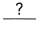Use theof powers property.
=Simplify.
67,

Explanation:
Given 64 • 63 using the Product of Powers Property states that when
multiplying two exponents with the same base, we add the exponents and
keep the base, So  64 • 63
= 64 + 3 = 67.

Question 2.
(-5) • (-5)5
(-5) • (-5)5 =Use theof powers property.
=Simplify the exponent.
=Simplify.
(-5)6,

Explanation:
Given (-5) • (-5)5 using the Product of Powers Property states that when
multiplying two exponents with the same base, we add the exponents and
keep the base, So  (-5) • (-5)5
=(-5) 1 + 5 = (-5)6.

Question 3.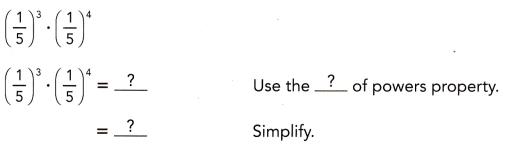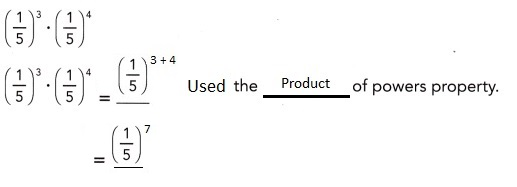Explanation:
Given $$\left(\frac{1}{5}\right)^{3}$$ • $$\left(\frac{1}{5}\right)^{4}$$
using the Product of Powers Property states that when
multiplying two exponents with the same base, we add the exponents and
keep the base $$\left(\frac{1}{5}\right)^{3}$$ • $$\left(\frac{1}{5}\right)^{4}$$ =
$$\left(\frac{1}{5}\right)^{3 +4}$$ = $$\left(\frac{1}{5}\right)^{7}$$.

Question 4.
p3 • p6
p3 • p6 =Use theof powers property.
=Simplify.
p3 • p6 = p3 + 6 = p9,

Explanation:
Given $${p}^{3}$$ • $${p}^{6}$$
using the Product of Powers Property states that when
multiplying two exponents with the same base, we add the exponents and
keep the base $${p}^{3}$$ • $${p}^{6}$$ =
$${p}^{3 + 6}$$ = $${p}^{9}$$.

Question 5.
(-c)4 • (-c)
(-c)4 • (-c)2 = (-c)4+2 = (-c)6,

Explanation:
Given $${-c}^{4}$$ • $${-c}^{2}$$
using the Product of Powers Property states that when
multiplying two exponents with the same base, we add the exponents and
keep the base $${-c}^{4}$$ • $${-c}^{2}$$ =
$${-c}^{4 + 2}$$ = $${-c}^{6}$$.

Question 6.
(3s)5 • (3s)
(3s)5 • (3s) = (3s)5+1 = (3s)6,

Explanation:
Given $${3s}^{5}$$ • $${3s}^{1}$$
using the Product of Powers Property states that when
multiplying two exponents with the same base, we add the exponents and
keep the base $${3s}^{5}$$ • $${3s}$$ =
$${3s}^{5 + 1}$$ = $${3s}^{6}$$.

Question 7.
pq3 • p5q2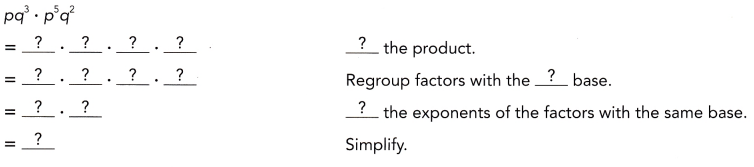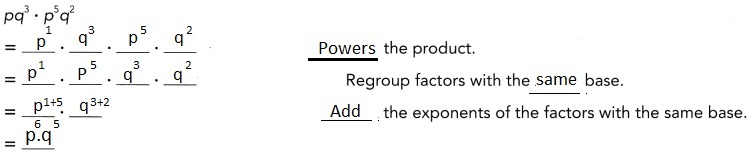Explanation:
Given $${p}{q}^{1}{3}$$ • $${p}{q}^{5}{2}$$
using the Product of Powers Property states that when
multiplying two exponents with the same base, we add the exponents and
keep the base $${p}^{1 + 5}$$ • $${q}^{3 + 2}$$ =
$${p}^{6}$$ • $${q}^{5}$$.

Question 8.
4s4t3 • 5s4t6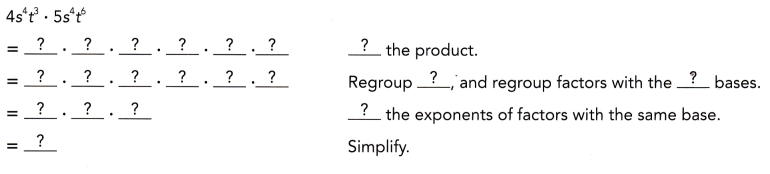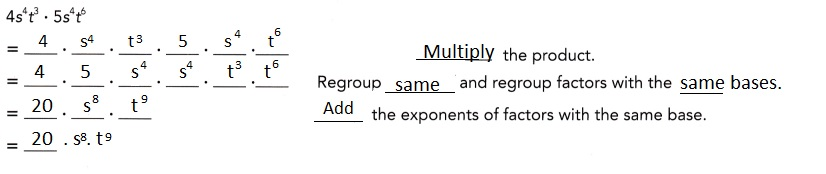Explanation:
Given 4. $${s}{t}^{4}{3}$$ • 5 . $${s}{t}^{4}{5}$$
using the Product of Powers Property states that when
multiplying two exponents with the same base, we add the exponents and
keep the base 20 . $${s}^{4+ 4}$$ •$${t}^{3 + 6}$$ =
20 . $${s}^{8}$$ • $${t}^{9}$$.

Question 9.
108 ÷ 105
108 ÷ 105 =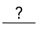Use theof powers property.
=Simplify.
108 ÷ 105 = 103,

Explanation:
Given to find 108 ÷ 105  dividing them with the exponent value in
the numerator (the top exponent) and subtract the exponent value of the
denominator (the bottom exponent). Here that means we take 108 ÷ 105 = 108-5= 103.

Question 10.
2.79 ÷ 2.76 =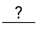=simplify
2.79 ÷ 2.76 = 2.79-6 = 2.73,

Explanation:
Given to find 2.79 ÷ 2.76 dividing them with the exponent value in
the numerator (the top exponent) and subtract the exponent value of the
denominator (the bottom exponent), means we take 2.79 ÷ 2.76 = 2.79-6= 2.73.

Question 11.
$$\left(\frac{5}{8}\right)^{6}$$ ÷ $$\left(\frac{5}{8}\right)$$

=Use theof powers property.
=Simplify.
$$\left(\frac{5}{8}\right)^{5}$$,

Explanation:
Given $$\left(\frac{5}{8}\right)^{6}$$ ÷ $$\left(\frac{5}{8}\right)$$
dividing them with the exponent value in
the numerator (the top exponent) and subtract the exponent value of the
denominator (the bottom exponent) means we take as
$$\left(\frac{5}{8}\right)^{6-1}$$ = $$\left(\frac{5}{8}\right)^{5}$$.

Question 12.
q7 ÷ q2
q7 ÷ q2 =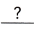=Simplify
$${q}^{5}$$,

Explanation:
Given to find q7 ÷  q2  dividing them with the exponent value in
the numerator (the top exponent) and subtract the exponent value of the
denominator (the bottom exponent). Here that means we take q7 ÷ q2 = q7-2= q5.

Question 13.
(-p)5 ÷ (-p)3
$${-p}^{2}$$,

Explanation:
Given to find (-p)5 ÷ (-p)2  dividing them with the exponent value in
the numerator (the top exponent) and subtract the exponent value of the
denominator (the bottom exponent). Here that means we take (-p)5 ÷ (-p)3 = (-p)5-3= (-p)2.

Question 14.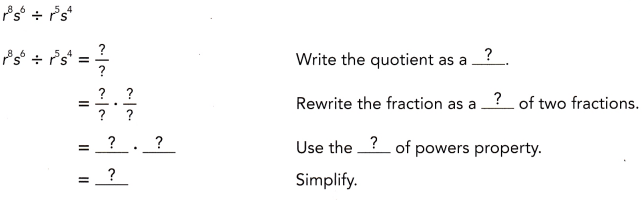(r)3 . (s)2,

Explanation:
Given to find (r)8 (s)6 ÷ (r)5 (s)4 dividing them with the exponent value in
the numerator (the top exponent) and subtract the exponent value of the
denominator (the bottom exponent). Here that means we take
(r)8 (s)6 ÷ (r)5 (s)4 = (r)8-5 . (s)6-4 = (r)3 . (s)2.

Question 15.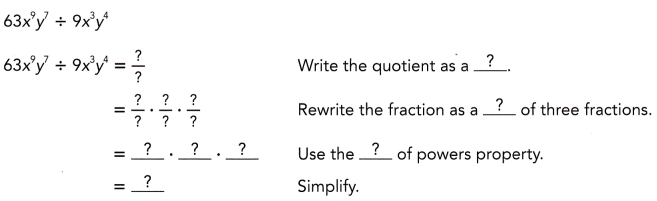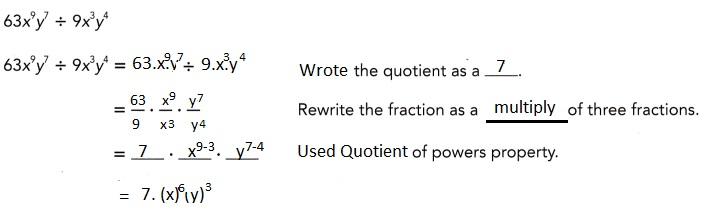Explanation:
Given to find 63 (x)9 (y)7 ÷ 9.(x)3 (y)4 dividing them with the exponent value in
the numerator (the top exponent) and subtract the exponent value of the
denominator (the bottom exponent). Here that means we take
63 (x)9 (y)7 ÷ 9 (x)3 (y)4 = 7 . (x)9-3 . (y)7-4 = 7. (x)6 (y)3 .

Question 16.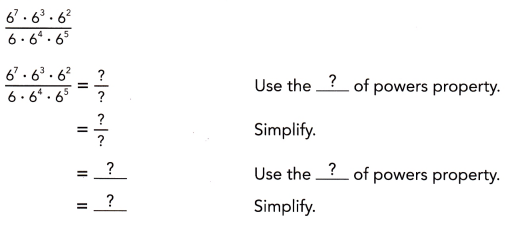$${6}^{2}$$,

Explanation:
Given to find (6)7 (6)3 (6)2 ÷ (6)1 (6)4 (6)5 dividing them with the exponent value in
the numerator (the top exponent) and subtract the exponent value of the
denominator (the bottom exponent). Here that means we take
(6)7 (6)3 (6)2 ÷ (6)1 (6)4 (6)5 = (6)7+3+2÷ (6)1+4+5 =
(6)12 ÷ (6)10=(6)12-10
= (6)2.

Question 17.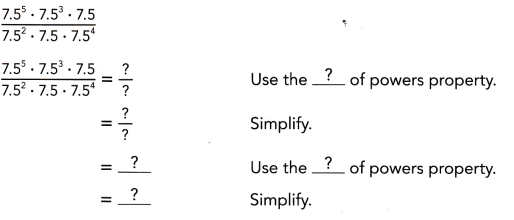$${7.5}^{2}$$,

Explanation:
Given to find (7.5)5 (7.5)3 (7.5)1 ÷ (7.5)2 (7.5)1 (7.5)4 dividing them with the exponent value in
the numerator (the top exponent) and subtract the exponent value of the
denominator (the bottom exponent). Here that means we take
(7.5)5 (7.5)3 (7.5)1 ÷ (7.5)2 (7.5)1 (7.5)4 = (7.5)5+3+1÷ (7.5)2+1+4 =
(7.5)9 ÷ (7.5)7=(7.5)9-7
= (7.5)2.

Question 18.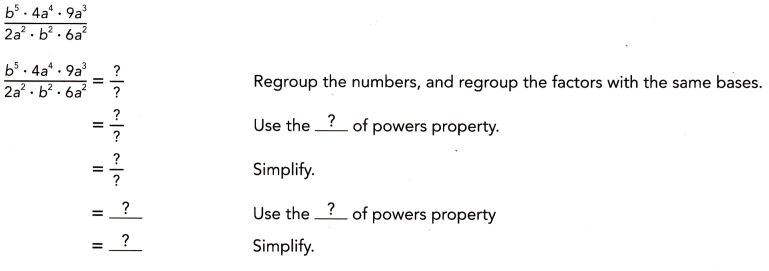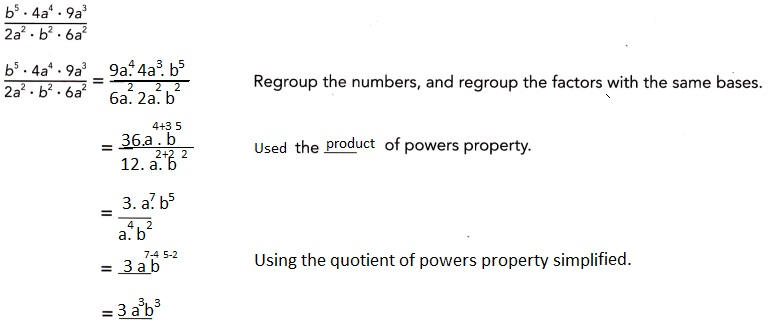Explanation:
Given to find (b)5 (4a)4 (9a)3 ÷ (2a)2 (b)2 (6a)2 dividing them with the exponent value in
the numerator (the top exponent) and subtract the exponent value of the
denominator (the bottom exponent). Here that means we take
(9).(4).(a)4+3.(b)5 ÷ (2).(6).(a)2+2 (b)3 = ((36).(a)7 .(b)5 )÷ (12). (a)4.(b)2 =
(3)(a)7-4 (b)5-2=(3)(a)3
(b)3.

Question 19.
Jupiter is approximately 108 kilometers from the Sun.
The dwarf planet Eris is about 101° kilometers from the Sun.
How many times as far as Jupiter is Eris from the Sun?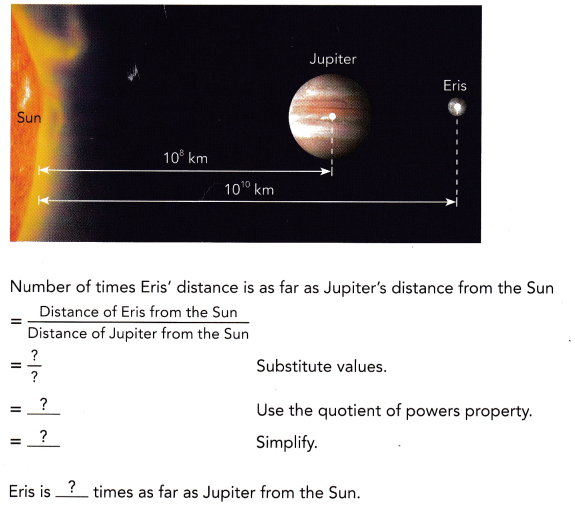Eris is 100 times as far as Jupiter from the Sun,

Explanation: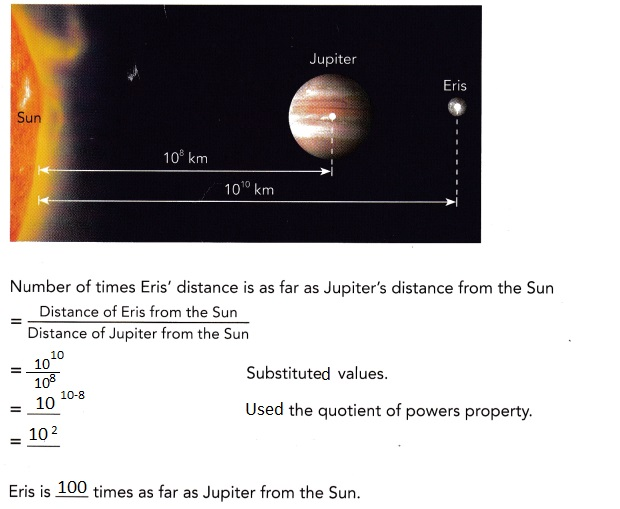### Math in Focus Course 3A Practice 1.2 Answer Key

Question 1.
(-2)6 • (-2)2
(-2)8 ,

Explanation:
Given (-2)6 • (-2)2 using the Product of Powers Property states that when
multiplying two exponents with the same base, we add the exponents and
keep the base, So  (-2)6 • (-2)2
=(-2) 6 + 2 = (-2)8.

Question 2.
7.23 • 7.24
7.23 . 7.24 = 7.23+4 = 7.27,

Explanation:
Given to find 7.23 . 7.24 using the Product of Powers Property states that when
multiplying two exponents with the same base, we add the exponents and
keep the base, So
7.23 . 7.24 = 7.23+4= 7.27.

Question 3.
105 • 104
109

Explanation:
Given 105 • 104 using the Product of Powers Property states that when
multiplying two exponents with the same base, we add the exponents and
keep the base, So 105 • 104 = 105+4 = 109.

Question 4.
$$\left(\frac{2}{3}\right) \cdot\left(\frac{2}{3}\right)^{5}$$
$$\left(\frac{2}{3}\right)^{6}$$,

Explanation:
Given $$\left(\frac{2}{3}\right)^{1}$$ • $$\left(\frac{2}{3}\right)^{5}$$
using the Product of Powers Property states that when
multiplying two exponents with the same base, we add the exponents and
keep the base $$\left(\frac{2}{3}\right)^{1}$$ • $$\left(\frac{2}{3}\right)^{5}$$ =
$$\left(\frac{2}{3}\right)^{1 +5}$$ = $$\left(\frac{2}{3}\right)^{6}$$.

Question 5.
p • p8
p1 • p8 = p1 + 8 = p9,

Explanation:
Given $${p}^{1}$$ • $${p}^{8}$$
using the Product of Powers Property states that when
multiplying two exponents with the same base, we add the exponents and
keep the base $${p}^{1}$$ • $${p}^{8}$$ =
$${p}^{1 + 8}$$ = $${p}^{9}$$.

Question 6.
q8 ÷ q
$${q}^{7}$$,

Explanation:
Given to find q8 ÷  q1  dividing them with the exponent value in
the numerator (the top exponent) and subtract the exponent value of the
denominator (the bottom exponent). Here that means we take q8 ÷ q1 = q8-1= q7.

Question 7.
xy2 • x4y3
$${x}^{5}$$ • $${y}^{5}$$,

Explanation:
Given xy2 • x4y3
using the Product of Powers Property states that when
multiplying two exponents with the same base, we add the exponents and
keep the base $${x}^{1 + 4}$$ • $${y}^{2 + 3}$$ =
$${x}^{5}$$ • $${y}^{5}$$.

Question 8.
2x2y4 • 5x5y
10 X $${x}^{7}$$ • $${y}^{5}$$,

Explanation:
Given 2x2y4 • 5x5y using the Product of Powers Property states that when
multiplying two exponents with the same base, we add the exponents and
keep the base (2 X 5) X $${x}^{2 + 5}$$ • $${y}^{4 + 1}$$ =
10 X $${x}^{7}$$ • $${y}^{5}$$.

Question 9.
2.5x3y6 • 3x2y4
7.5 X $${x}^{5}$$ • $${y}^{10}$$,

Explanation:
Given 2.5 X x3y6 • 5x2y4 using the Product of Powers Property states that when
multiplying two exponents with the same base, we add the exponents and
keep the base (2.5 X 3) X $${x}^{3 + 2}$$ • $${y}^{6 + 4}$$ =
7.5 X $${x}^{5}$$ • $${y}^{10}$$.

Question 10.
(-3)4 ÷ (-3)2
(-3)2 ,

Explanation:
Given to find (-3)4 ÷  (-3)2  dividing them with the exponent value in
the numerator (the top exponent) and subtract the exponent value of the
denominator (the bottom exponent). Here that means we take (-3)4 ÷ (-3)2 = (-3)4-2= (-3)2.

Question 11.
210 ÷ 25
25,

Explanation:
Given to find 210 ÷  25  dividing them with the exponent value in
the numerator (the top exponent) and subtract the exponent value of the
denominator (the bottom exponent). Here that means we take 210 ÷ 25 = 210-5= 25.

Question 12.
$$\left(-\frac{1}{6}\right)^{5} \div\left(-\frac{1}{6}\right)^{2}$$
$$\left(-\frac{1}{6}\right)^{3}$$,

Explanation:
Given $$\left(-\frac{1}{6}\right)^{5}$$ ÷ $$\left(-\frac{1}{6}\right)^{2}$$
dividing them with the exponent value in
the numerator (the top exponent) and subtract the exponent value of the
denominator (the bottom exponent) means we take as
$$\left(-\frac{1}{6}\right)^{5-2}$$ = $$\left(-\frac{1}{6}\right)^{3}$$.

Question 13.
63y3z5 ÷ 9
7y3z5,

Explanation:
Given to find 63 (y)3 (z)5 ÷ 9 dividing them with the exponent value in
the numerator (the top exponent) and subtract the exponent value of the
denominator (the bottom exponent). Here that means we take
63 (y)3 (z)5 ÷ 9 = 7. (y)3 (z)5 .

Question 14.
h2k5 ÷ hk4
hk,

Explanation:
Given to find  (h)2 (k)5 ÷ h.(k)4 dividing them with the exponent value in
the numerator (the top exponent) and subtract the exponent value of the
denominator (the bottom exponent). Here that means we take
(h)2 (k)5 ÷  h. (k)4 = (h)2-1 . (k)5-4 = hk.

Question 15.
64a8b5 ÷ 4a3b2
16a5b3,

Explanation:
Given to find 64 (a)8 (b)5 ÷ 4.(a)3 (b)2 dividing them with the exponent value in
the numerator (the top exponent) and subtract the exponent value of the
denominator (the bottom exponent). Here that means we take
64 (a)5 ( b)3 ÷ 4 (a)3 (b)2 = 16 . (a)8-3 . (b)5-2 = 16. a5b3

Question 16.
$$\frac{5^{9} \cdot 5^{7} \cdot 5^{8}}{5^{3} \cdot 5^{2} \cdot 5}$$
518,

Explanation:
Given to find (5)9 (5)7 (5)8 ÷ (5)3 (5)2 (5)1 dividing them with the exponent value in
(5)9 (5)7 (5)8 ÷ (5)3 (5)2 (5)1 the numerator (the top exponent) and
subtract the exponent value of the denominator (the bottom exponent).
Here that means we take (5)9+7+8 ÷ (5)3+2+1 = (5)24 ÷ (5)6=(5)24-6
= (5)18.

Question 17.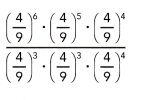$$\left(\frac{4}{9}\right)^{5}$$,

Explanation:
Given to find $$\left(\frac{4}{9}\right)^{6}$$. $$\left(\frac{4}{9}\right)^{5}$$.
$$\left(\frac{4}{9}\right)^{4}$$  ÷ $$\left(\frac{4}{9}\right)^{3}$$. $$\left(\frac{4}{9}\right)^{3}$$. $$\left(\frac{4}{9}\right)^{4}$$ dividing them with the exponent value in
the numerator (the top exponent) and subtract the exponent value of the
denominator (the bottom exponent). Here that means we take
$$\left(\frac{4}{9}\right)^{6+5+4}$$ ÷ $$\left(\frac{4}{9}\right)^{3 +3+4}$$,
$$\left(\frac{4}{9}\right)^{15}$$ ÷ $$\left(\frac{4}{9}\right)^{10}$$,
$$\left(\frac{4}{9}\right)^{15-10}$$ = $$\left(\frac{4}{9}\right)^{5}$$.

Question 18.
$$\frac{a^{9} \cdot a^{2} \cdot a^{3}}{a^{6} \cdot a^{3} \cdot a^{4}}$$
a,

Explanation:
Given $$\frac{a^{9} \cdot a^{2} \cdot a^{3}}{a^{6} \cdot a^{3} \cdot a^{4}}$$
to find dividing them with the exponent value in
the numerator (the top exponent) and subtract the exponent value of the
denominator (the bottom exponent). Here that means we take
$$\frac{a^{9+2+3} \cdot}{a^{6+3+4} \cdot}$$ =
$$\frac{a^{14} \cdot}{a^{13} \cdot}$$ = (a)14-13 = = a.

Question 19.
$$\frac{b^{4} \cdot b^{6} \cdot b}{b^{3} \cdot b^{3} \cdot b^{3}}$$
(b)2,

Explanation:
Given $$\frac{b^{4} \cdot b^{6} \cdot b}{b^{3} \cdot b^{3} \cdot b^{3}}$$
to find dividing them with the exponent value in
the numerator (the top exponent) and subtract the exponent value of the
denominator (the bottom exponent). Here that means we take
$$\frac{b^{4+6+1} \cdot}{a^{3+3+3} \cdot}$$ =
$$\frac{b^{11} \cdot}{b^{9} \cdot}$$ = (b)11-9 = (b)2.

Question 20.
$$\frac{3 x^{3} \cdot z^{4} \cdot 4 x^{3}}{2 x \cdot x \cdot 3 z}$$
2x4z3,

Explanation:
Given to find 3.(x)3 (z)4 . 4(x)3 ÷ 2.(x)(x) (z) dividing them with the exponent value in
the numerator (the top exponent) and subtract the exponent value of the
denominator (the bottom exponent). Here that means we take
12 (x)6 (z)4 ÷ 6 (x)2 (z) = 2 . (x)6-2 . (z)4-1 = 2. (x)4 (z)3 .

Question 21.
$$\frac{4 c^{6} \cdot 3 b^{4} \cdot 9 c^{5}}{b^{3} \cdot 6 c^{3} \cdot 2 c^{3}}$$
9bc5,

Explanation:
Given to find $$\frac{4 c^{6} \cdot 3 b^{4} \cdot 9 c^{5}}{b^{3} \cdot 6 c^{3} \cdot 2 c^{3}}$$
dividing them with the exponent value in the numerator (the top exponent) and
subtract the exponent value of the denominator (the bottom exponent).
Here that means we take 4(c)6. 3(b)4 . 9(c)÷ (b)3 . 6 (c)3 . 2(c)=
9 . (b)4-3 . (c)11-6 = 9.(b).(c)5 .

Question 22.
Pluto has a diameter of about 103 kilometers. The diameter of Saturn is
approximately 105 kilometers.
How many times as great as Pluto’s diameter is Saturn’s diameter?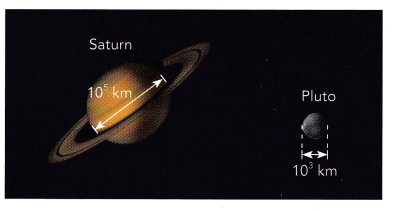100 times as great as Pluto’s diameter is Saturn’s diameter,

Explanation:
Given Pluto has a diameter of about (10)3 kilometers.
The diameter of Saturn is approximately (10)5 kilometers.
So number of times as great as Pluto’s diameter is Saturn’s diameter is
(10)5-3 = (10)2,
therefore 100 times as great as Pluto’s diameter is Saturn’s diameter.

Question 23.
Use the rectangular prism shown.
a) Express the volume of the rectangular prism using exponential notation.
6(x)3,

Explanation:
Given width of the rectangular prism as 2x units,
height as x units and length as 3x units so the
volume of the rectangular prism using exponential notation is
w X h X l = 2x X x X 3x =6(x)1+1+1 = 6(x)3.

b) Another prism has dimensions that are twice the dimensions of the prism shown.
Express the volume of that prism using exponential notation.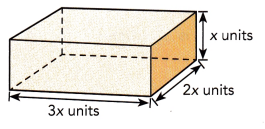Explanation:
Given another prism has dimensions that are twice the dimensions of the prism shown.
Expressing the volume of that prism using exponential notation is
width of the rectangular prism as 2x X 2x = 4x1+1  = 4x2 units,
height as 2 X x = 2x units and length as 3x X 3 x = 9x1+1 = 9x2 units,
So the volume of the rectangular prism using exponential notation is
w X h X l = 4x2 X 2x X 9x2 = 72 (x)2+1+2 = 72(x)5.

c) How many times greater is the volume of the larger prism than the volume of the smaller prism?